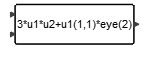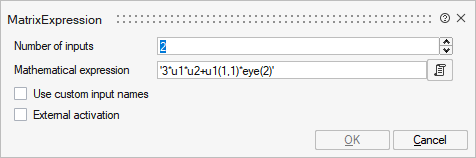# MatrixExpression

The output of this block is a matrix obtained by evaluating the matrix expression specified as a block parameter. The expression specifies the output in terms of input matrices u1,...un where ui is the ith matrix input (except if using custom input names), matrix parameters (defined in the context of the diagram), and constants. Only real matrices are supported.## Library

Activate/MatrixOperations

## Description

The output of this block is a matrix obtained by evaluating the matrix expression specified as block parameter. The expression specifies the output in terms of input matrices u1,...un where ui is the ith matrix input (except if using custom input names), matrix parameters (defined in the context of the diagram) and constants.

Only real matrices are supported. OML functions can be used in the mathematical expression, as long as the value of the function can be determined in the evaluation phase.

Supported syntax is listed as following

Arithmetical operator: + - * / ^

Logical operator: | & ! ~

Relational operator: > < >= <= == ~= <>

Conditional operators: Element-wise if expression, Element-wise switch expression

Matrix operators: [] , ; (,) () : \$ :=

Elementary functions: abs exp log log10 min max sign sqrt hypot

Trigonometric functions: acos acosh asin asinh atan atan2 atanh cos cosh sin sinh tan

Rounding functions: ceil floor int round

Matrix functions: eye ones zeros det expm inv logm max min size sum svd

General math functions: rem power pow

For more details, please refer to the section about Matrix Expression in the Extended Definitions for Advanced Users.

## ParametersNameLabelDescriptionData TypeValid Values

nin

Number of inputs

Number

work

Workspace Evaluation

Hidden parameter used by the block to indicate available environemnt variables.

used_names

Used names

Hidden parameter used by the block to indicate used environment variables.

exx

Mathematical expression

String

useinputnames

Use custom input names

Number

0
1

inputnames

Custom input names

The variable names of inputs.

Structure

inputnames/inputname

Variable name

Input variable name.

Cell of strings

externalActivation

External activation

Number

0
1

## Ports

NameTypeDescriptionIO TypeNumber

Port 1

explicit

output

1

Port 2

explicit

input

nin

Port 3

activation

input

externalActivation

NameValueDescription

always active

no

direct-feedthrough

yes

zero-crossing

no

mode

no

continuous-time state

no

discrete-time state

no